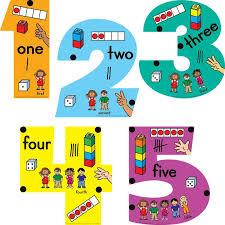# Determine 2860

Five natural numbers are given, each of which is a triple of the previous one. The largest number is bigger by 1152 than the middle number in this series. From these five numbers, determine the first number.

a =  16

### Step-by-step explanation:

b=3a
c=3b
d=3c
f=3d
f=1152+c

b=3·a
c=3·b
d=3·c
f=3·d
f=1152+c

3a-b = 0
3b-c = 0
3c-d = 0
3d-f = 0
c-f = -1152

Row 5 - 1/3 · Row 3 → Row 5
3a-b = 0
3b-c = 0
3c-d = 0
3d-f = 0
0.33333d-f = -1152

Row 5 - 0.33333333/3 · Row 4 → Row 5
3a-b = 0
3b-c = 0
3c-d = 0
3d-f = 0
-0.88889f = -1152

f = -1152/-0.88888889 = 1296
d = 0+f/3 = 0+1296/3 = 432
c = 0+d/3 = 0+432/3 = 144
b = 0+c/3 = 0+144/3 = 48
a = 0+b/3 = 0+48/3 = 16

a = 16
b = 48
c = 144
d = 432
f = 1296

Our linear equations calculator calculates it.Did you find an error or inaccuracy? Feel free to write us. Thank you!

Tips for related online calculators
Do you have a linear equation or system of equations and looking for its solution? Or do you have a quadratic equation?